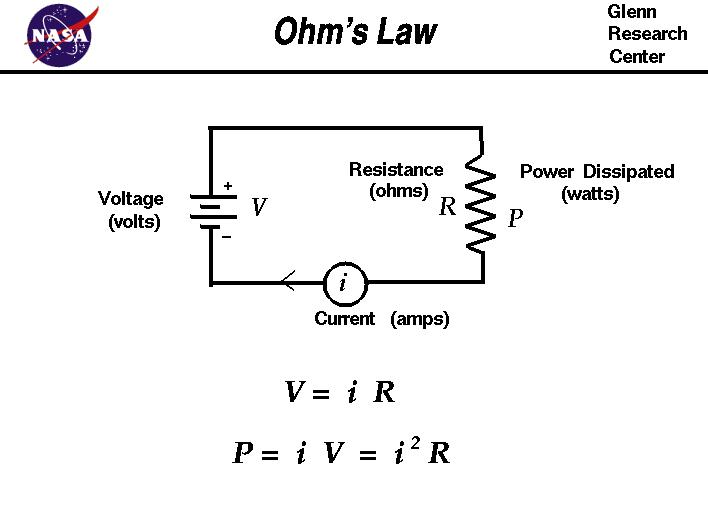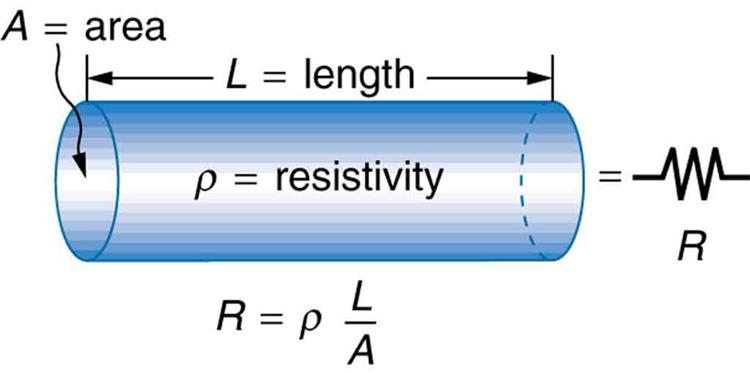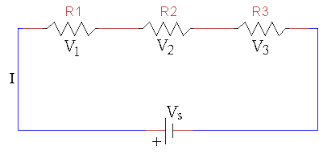Electricity is a usual term that is used for all phenomena caused by electric charge whether it may be static or, dynamic. By electrical network we mean it is a collection of all types of Electric Elements such as resistances, capacitances, inductive coils, and power supply which are connected jointly to produce several circuits which are inter-related nature for the specified requirements.

## Ohm's Law

When a certain potential difference or voltage is applied across a conductor, current will flow through the conductor. The great German scientist Dr. George Simon Ohm discovered a definite relationship between applied voltage and current, which was considered afterward as Ohm's law.Ohm's law states that the electric current flowing through a conductor material is directly proportional to the voltage difference between the ends of the conductor material. keeping the physical conditions unaltered.

Let us take the voltage difference or potential difference between the ends of the conductor material be 'V' and the current flowing through it be 'T', then according to Ohm's law.

Ohm's law holds good where temperature, pressure, and other physical conditions do not change. Ohm's law does not hold good for electrolytes, arc lamps, semiconductors, vacuum tube radio valves, gas-filled tubes, transistors, etc.

## Law of Resistance

This law states that the resistance of conductor material is directly proportional to the length of the conductor, inversely proportional to the cross-section of the conductor, and depends upon the nature of the conductor material at a constant temperature.

Now we can say that,

Where a = cross-sectional area of the conductor material.

\ R = resistance of the conductor material.
l = length of the conductor material.
p = specific resistance or resistivity of the conductor material.

### Factors in Which the Resistance of a Conductor Depends:

The following are the factors on which the resistance of a conductor depends:

(1) Length of the Substance: The resistance of the conductor directly increases with the length of the substance.

(2) Cross-Sectional Area of the Substance (A): The resistance of the conductor is inversely proportional to the C. S. area of the substance. If the C.S. area increases the resistance will be decreased.

(3) Material Used in the Substance: The resistance of the conductor varies with the material used.

(4) Working Temperature: Resistance of the conductor (pure metals and alloys) increases with the rise of temperature but in the case of Insulators, semi-conductors & electrolytes decrease the resistance with the rise of temperature.

(5) Nature of Current Used: Due to the skin effect, the resistance of the material will be higher when alternating current flows than that of the flow of direct current (nearly about 15% to 20%).

(6) Other Factors: In some specified materials the resistance will depend upon magnetic fields, and light. pressure and whatnot. Bismuth shows higher resistance when placed in a magnetic field When the semiconductor (selenium) is kept in light energy, its resistance will be lower.

## Resistivity or, Specific Resistance

The resistivity of a conductor material may be stated as the resistance offered by the unit length of the material and having a unit cross-section of the materialWhere d = diameter of the material in meters. material's resistivity may also be stated as also the resistance offered between the opposite faces of a unit cube of the material. It is denoted by 'p' (Rho) and the unit used (in S. I) is ohm- m.

We know

### Effect of Temperature on the Resistance

(1) In the case of pure metal, that is copper, silver, gold, etc, with the rise of temperature. the resistance will increase regularly.

(2) In the case of an alloy i.e., Bronze, Babbit metal, etc. with the rise of temperature, the resistance will increase irregularly.

(3) In the case of insulators, semi-conductor, and electrolytes, with the temperature rise, the resistance will decrease.

## Temperature-Co-efficient of Resistance

The temperature-co-efficient of resistance of conductor material is the change in resistance of the material per ohm per degree change in temperature, counting from 0°C is denoted by an (alpha).

Again, the temperature-co-efficient of resistance can be found in the following formula:

## Resistance in Series

When the resistance of the conductor is joined end to end, they are called Series grouping.Let R1, R2 and R3 are connected in Series and V1, V2 and V3 be the voltage drops in these corresponding resistances and circuit-current is '1', which is constant in the series circuit:

## Resistance in Parallel

If the similar ends of each resistance are joined to a common point, and at the other ends, other resistors are joined to another common point, then it is said to be a parallel grouping of resistances.